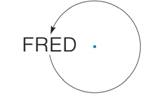Chapter 2.6, Problem 22EElementary Geometry For College St...

7th Edition
Alexander + 2 others
ISBN: 9781337614085

Solutions

Chapter
SectionElementary Geometry For College St...

7th Edition
Alexander + 2 others
ISBN: 9781337614085
Textbook Problem

What word is produced by 360 ° rotation about the point?To determine

To find:

The word produced by 360° rotation about the point.

Explanation

Given:

The given figure is,

Figure (1)

Definition Used:

Rotation of the word about 360° will create a full circle.

Approach:

Consider a reference point A on the word for rotation of word.

Rotate the word by some degrees at a time till a circle is created by rotation.

The rotation of figure is given below.

Still sussing out bartleby?

Check out a sample textbook solution.

See a sample solution

The Solution to Your Study Problems

Bartleby provides explanations to thousands of textbook problems written by our experts, many with advanced degrees!

Get Started

Evaluate the integrals in Problems 7-36. Check your results by differentiation. 8.

Mathematical Applications for the Management, Life, and Social Sciences

In Exercises 73-80, find the indicated limits, if they exist. 74. limx4x21x+2

Applied Calculus for the Managerial, Life, and Social Sciences: A Brief Approach

Simplify: 90

Elementary Technical Mathematics

Find the derivative of the function. s(t)=1+sint1+cost

Single Variable Calculus: Early Transcendentals, Volume I

In Exercises 1728, use the logarithm identities to obtain the missing quantity.

Finite Mathematics and Applied Calculus (MindTap Course List)

Find the following products. (4+7i)2

Trigonometry (MindTap Course List)

Sometimes, Always, or Never: If for all n and both {an} and {cn} converge, then{bn} converges.

Study Guide for Stewart's Single Variable Calculus: Early Transcendentals, 8th